# Quarantine cupcakes

Mr. Honse was baking quarantine cupcakes. Mrs. Carr made twice as many as Mr. Honse. Ms. Sanchez made 12 cupcakes more than Mr. Honse. If they put all their cupcakes together (which they can’t because. .. quarantine!) they would have 108 cupcakes. How may cupcakes did each Math teacher make?

Correct result:

C =  48
H =  24
S =  36

#### Solution:

C=2•H
S = 12+H
C+H+S = 108

C-2H = 0
H-S = -12
C+H+S = 108

C = 48
H = 24
S = 36

Our linear equations calculator calculates it.We would be pleased if you find an error in the word problem, spelling mistakes, or inaccuracies and send it to us. Thank you!Math student
the quarantine cookie problem is very timely. am making my learning module and i took it as a sample problem. thanksMath student
i mean cupcakes ha haTips to related online calculators
Do you have a linear equation or system of equations and looking for its solution? Or do you have quadratic equation?

## Next similar math problems:

• Two math problems1) The sum of twice a number and -6 is nine more than the opposite of that number. Find the number. 2) A collection of 27 coins, all nickels, and dimes, is worth \$2.10. How many of each coin are there? The dime, in United States usage, is a ten-cent coin.
• Three friendsCuba, Matthew and their friend Adam found a brigade during their weekend weekends because they wanted to make a joint trip to the Alps that they planned for the spring break. Cuba enjoyed the skiing trip very much, so he was not lazy to get up and went to
• Sweets, candyGrandfather gave out sweets to four children. At the last moment, two more children came, so in order to have them all the same, each of the four children would receive four candies less than they would have received if they had not. How much did my grand
• Three friends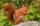Three friends squirrels together went to collect hazelnuts. Zrzecka he found more than twice Pizizubka and Ouska even three times more than Pizizubka. On the way home they talked while eating and was cracking her nuts. Pizizubka eaten half of all nuts whi
• Candy and boxesWe have some number of candy and empty boxes. When we put candies in boxes of ten, there will be 2 candies and 8 empty boxes left, when of eight, there will be 6 candies and 3 boxes left. How many candy and empty boxes left when we put candies in boxes of
• Average monthly salaryA total of 10 teachers work at one small school in Moravia. The monthly salary of each is 21,500 CZK or 21,800 CZK or 22,500 CZK according to their education and age. The average monthly salary for this school's teacher is 21 850 CZK. How many teachers of
• Euler problem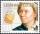Someone buys a 180 tolars towels. If it was for the same money of 3 more towels, it would be 3 tolars cheaper each. How many were towels?
• Chickens and rabbitsIn the yard were chickens and rabbits. Together they had 27 heads and 86 legs. How many chickens and how many rabbits were in the yard?
• Lookout towerHow high is the lookout tower? If each step was 3 cm lower, there would be 60 more of them on the lookout tower. If it was 3 cm higher again, it would be 40 less than it is now.
• SubjectsStudent makes a mathematical task for five minutes and the task from biology in twelve minutes. Another day he have homework in math and biology which consists of eleven tasks together. To do the job took hour and a half. How many tasks maked in each subj
• PoolIf water flows into the pool by two inlets, fill the whole for 19 hours. The first inlet filled pool 5 hour longer than the second. How long pool take to fill with two inlets separately?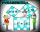Two fifth-graders teams competing in math competitions - in Mathematical Olympiad and Pytagoriade. Of the 33 students competed in at least one of the contest 22 students. Students who competed only in Pytagoriade was twice more than those who just competePeter and Paul together have 26 years. Four years ago, Paul was twice older than Peter. How much is Paul and how much Peter?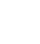Simiyu and Nasike each collected a number of stones in an arithmetic lesson. If Simiyu gave Nasike 5 stones, Nasike would have twice as many stones as Simiyu. If initially, Simiyu had five stones less than Nasike how many stones did each have?In the competition, 10 contestants answer five questions, one question per round. Anyone who answers correctly will receive as many points as the number of competitors answered incorrectly in that round. One of the contestants after the contest said: We g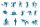Three scouts went on a three day trip. The second day went 4 km more than the first day. The third day went two times less than the first day. They went along 54 km. How many kilometers went every day together?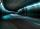The highway tunnel was built from two ends in the north-south direction. The average daily performance of the "northern party" rasters was higher than the average daily power of the "southern party" of the rasters. After 55 business days, both parties met